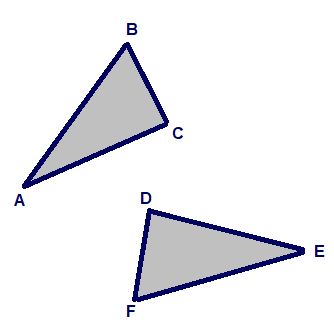# Based on the diagram above, is the following statement true or false? BCA is congruent to FDE.

## Question:Based on the diagram above, is the following statement true or false?

∠BCA is congruent to ∠FDE.

## Congruency in Triangles

Two triangles are considered to be similar if the ratios of the corresponding sides are equal. If the side lengths of all the sides are equal for both the triangles and they are considered to be congruent. Two triangles are congruent if they are superimposable on one another. We can prove congruency by many theorems but the most basic of them is the SSS theorem which stands for the side-side-side theorem which basically means that if the corresponding sides of a triangle are equal then the two triangles are congruent.

## Answer and Explanation: 1

Since no metric is given and we are not sure if the corresponding sides and the included angle are equivalent, we cannot prove congruency.

However, if the following statements are true then the congruency holds.

{eq}BC = FD\\ CA = DE\\ BA = FE {/eq}

We can also prove congruency if any two sides and the included angle are equivalent. For example: This is the case when:

{eq}BC = FD\\ \angle C = \angle D\\ CA = DE\\ {/eq}

Thus, the two triangles can be proved to be congruent if these conditions are met.